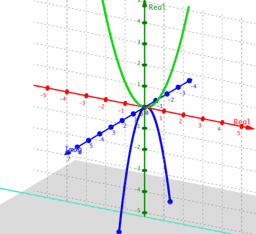# Phantom Graphs

Phantom graphs use the 3D graphing capability of GeoGebra to show all solutions, both real and complex, to many equations. In general, they will show all solutions to f(x)=c where c ranges over all reals. Explore to find that by extending to complex numbers we can graph the solutions to x^2=-4 and even see that cos(z)=7 actually has a complex solution (an infinite number of them). In all the applets in this book if the point A(a, b, c) is on the phantom graph, then letting x=a+ic and y=b will give values that satisfy the original equation. Note that y=b is the middle value since we are using the x-axis as real and the z-axis as the imaginary axis.